top of page

### Pathfinding Algorithms for DEX Aggregation and Smart Order Routing

##### Introduction

This article outlines the ongoing research Deeplink is undertaking regarding the framing of both smart order routing and DEX aggregation as pathfinding problems. A brief overview of what pathfinding is and some common techniques are provided, along with a detailed explanation of how this field of study applies to these problems, and finally, a collection of related works are investigated.

##### What is Pathfinding?

Pathfinding is the computational field of identifying the shortest route between two points. Pathfinding algorithms are used across many industries and applications including search engines, video games, GPS navigation software, and robotics, to name a few.

One of the simplest examples to consider is a GPS route optimization algorithm in which nodes are landmarks or destinations, and edges are the paths between those locations, weighted by the physical distances, a pathfinding algorithm could find the shortest route through these locations from one location to another by traversing the edges in such a way that minimizes the total weight traversed.

Another example, reminiscent of Euler’s Königsberg Bridges problem, can be found in infrastructure planning such as logistics, motorway, or railway design. For instance, when designing complex systems such as a metro, it is important to minimize redundant travel in the layout of the train lines in order to avoid congestion and slow journeys. One fundamental approach to doing this is to use pathfinding algorithms to identify the shortest Euler path between all relevant metro stations, in other words, the shortest path which visits each station at least once. A system of stations can also be broken down into discrete lines, each of which can have its own shortest Euler path. This can be seen in the following representation courtesy of Paragon Routing, a pathfinding and route optimization software provider.Pathfinding Algorithms for DEX Aggregation and Smart Order Routing
Introduction
What is Pathfinding?
Glossary
Smart Order Routing
DEX Aggregation
Graphs

#### Glossary

In previous articles we have outlined both smart order routing and DEX aggregation in some depth, to fully grasp the necessity for pathfinding algorithms in enhancing these spaces, we recommend reading those articles. However, a brief summary of each will also be provided here, along with a few important preliminary concepts.

##### Smart Order Routing

Smart order routing (SOR) is an automated process in which orders on exchanges are handled with the intent of attaining the most desirable path across trading venues. In a DEX, this generally takes the form of finding the optimal path of swaps across a set of liquidity pools in order to take advantage of the liquidity depth of those pools, and mitigate the effects of fragmented liquidity. The primary cause for concern regarding this liquidity fragmentation being negative slippage, losses incurred on account of a change in spot price in the time between an order being placed and executed.

##### DEX Aggregation

A DEX is a trading venue consisting of a set of liquidity pools that facilitate the exchange of assets without a central authority or the need for users to forfeit custody over their assets. DEXs are prone to the aforementioned issues of liquidity fragmentation as their asset pairs are segmented into liquidity pools, and as more and more venues arise, the overall market liquidity becomes more and more thinly spread.

DEX aggregators expose traders to more liquidity than any one DEX could provide by aggregating and connecting the services of multiple DEXs. A DEX aggregator can be thought of analogously to services like Expedia or Google Flights, which aggregate offerings from numerous airlines into one comparative service, giving users access to the best possible options for their needs.

##### Graphs

In computer science, pathfinding is most commonly represented using graphs-sets of connected nodes. In a graph, nodes can be thought of as places, computers, people, etc. depending on the application, while the connecting edges can be thought of as whatever relates those nodes to one another, e.g. an edge connecting two nodes representative of cities may be representative of a highway, or an edge connecting two asset pools may represent a swap between those two pools.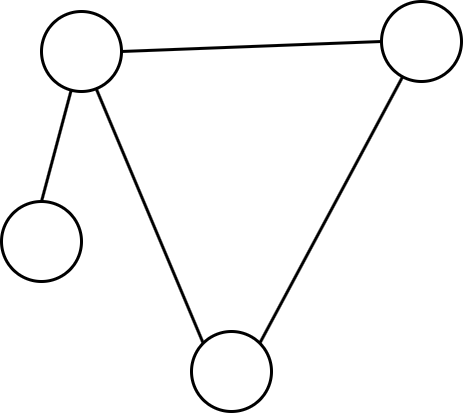Weighted Graphs

It is worth noting that there are many other representations of spaces which can be used in pathfinding, such as grids, 2D or 3D spaces, or even more abstract data structures such as octrees and quadtrees. However, graphs are the most widely used and most easily related to our use case.

##### Weighted Graphs

Graph edges can also be weighted, attributing values to the connections between nodes. For instance, the weight in the two cities example may be the physical distance between the cities, or in the asset pools example, the weight may indicate the fees incurred by making that swap.Directed Graphs
##### Directed Graphs

Additionally, graphs can be directed, that is, some or all edges can only be traversed in one direction. That is, a node can point to another node without that node pointing back to it. For example, if the only path between points A and B is a unidirectional road, that would be a directed edge connecting those two nodes.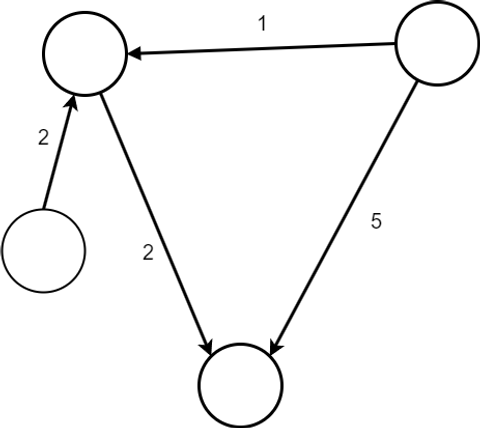Temporal Graphs

However, some nodes may still be traveled to from one another in a directed graph, this is represented by two arrows pointing from each node to the other. Furthermore, nodes can also point at themselves, indicating that it is a valid move to travel from that node to itself — this is known as a loop.

##### Temporal Graphs

Temporal graphs represent nodes and connections which change over time. For example, a node may only be accessible temporarily, or the weight of an edge may not be constant over time. Temporal graphs are a relatively recent development in graph theory, and can dramatically increase the complexity of tasks such as graph traversal. Temporal graphs are somewhat of an emerging field and are quite complex in nature, for further reading on the topic, here is a good resource that explores them in depths beyond the scope of this article.Graph Traversal
Metrics and Nuances of Pathfinding Algorithms
Informed vs. Uninformed Algorithms
Completeness
Termination
Big O Notation
Common Pathfinding Algorithms
Rapidly-exploring Random Trees

##### Graph Traversal

When representing spaces as graphs, pathfinding can be represented as graph traversals. This often involves finding the shortest path to connect two or more points, by using the least edges, or by accumulating the least weight along the paths connecting those nodes. Alternatively, graph traversal can find a path that visits each node in a graph in such a way that similarly minimizes the cost of doing so.

##### Metrics and Nuances of Pathfinding Algorithms

The following section outlines some terms and concepts which are useful in understanding the effectiveness or applicability of a given pathfinding algorithm.

##### Informed vs. Uninformed Algorithms

Informed algorithms (such as greedy search) have some information regarding their own goal, provided by the function which is used to estimate the closeness of its current state to its goal state (destination node, in our case).

Conversely, uninformed algorithms (such as depth-first and breadth-first search) do not have information regarding their own goal.

##### Completeness

A pathfinding algorithm is considered complete if it is guaranteed to complete execution, i.e. it will not get stuck running forever.

##### Termination

A pathfinding algorithm can have one or more termination conditions, requirements by which it will cease execution, such as finding the solution if it exists.

A pathfinding algorithm is admissible if an optimal solution is guaranteed to be found, provided enough time, memory, and computation are provided.

##### Big O Notation

Big O notation is the standard mathematical notation for describing the behavior of a function or algorithm as a limit when a relevant variable approaches a specific value such as infinity. It is used to express the efficiency of the algorithm, for example, we can say that the Bellman-Ford equation takes O(VE) time, meaning that we can expect a given graph with V vertices (nodes) and E edges to be solved by the Bellman-Ford algorithm in V*E steps.

#### ​

Pathfinding algorithms can largely be divided into one of three main categories, dynamic, greedy, or heuristic. This section will outline the differences between these approaches and provide some famous examples of each.

##### Rapidly-Exploring Random Trees

Rapidly exploring random tree (RRT) can be either greedy or dynamic depending on how the specific implementation is tweaked. RRT search is useful for exploring high-dimensional spaces, particularly, it is used to find open-loop trajectories in nonlinear systems. As such, it may be useful in the event that simple graphs do not adequately represent the complexity of the system at hand. RRT achieves its traversal by extending random branches into the largest unexplored regions of a given space; given enough time, it will give a relatively optimal path to the desired location. It can also be useful when the exact location of the goal object is not known. It is an industry-standard algorithm for pathfinding and route optimization in autonomous vehicles and robotics, as can be seen in this demonstration created by the author of this article:

Greedy Algorithms
Djikstra

RRT SLAM (simultaneous localization and mapping) Demonstration, source: Jack Ryan Lodge

The blue lines on the right-hand window depict the branches of the robot’s RRT-based pathfinding algorithm as it explores the building.

##### Greedy Algorithms

A greedy algorithm is one that builds a solution piece by piece, at each step it chooses the option which yields the most immediate benefits, in other words, the ‘greedy’ option. With greedy algorithms, there is no guarantee that an optimal solution will ever be found, though they are generally more efficient regarding memory usage and time complexity than their counterparts.

##### Djikstra

Djikstra’s algorithm is a simple yet effective greedy algorithm for finding the shortest path between one node and all other nodes in a graph, or it can be used to give the shortest distance between any two given nodes. It essentially calculates the distance of each node one-by-one from a given source node, updating a list of shortest paths each time a longer path is undercut. Many other algorithms have built on this concept but retain the principle of iteratively searching for near-optimal paths and replacing them once better ones are identified until the most optimal path is found.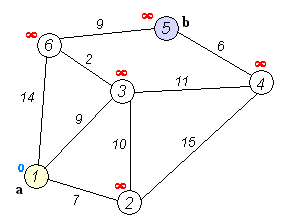A*
##### A*

A* builds on Djikstra’s algorithm by introducing a heuristic function that can be used to prioritize nodes, in other words, it is an informed algorithm. Heuristics can dramatically improve the performance of A* if the problem facilitates such an optimal heuristic, and the heuristic is optimally designed. Similarly to Djikstra’s algorithm, A* begins at a source node, it then aims to create a path to a destination with the smallest possible cost by creating and minimizing a tree of paths between source and destination nodes until the terminal criteria are reached — The shortest of these paths is then selected.Dynamic Algorithms
Bellman-Ford

A* Algorithm

##### Dynamic Algorithms

When solving parts of a problem (sub-problems), dynamic algorithms store the solutions to those sub-problems along the way then make decisions at each step based on the current situation and the solution to previously solved sub-problems when calculating for optimal solutions. Unlike greedy algorithms, dynamic algorithms are guaranteed to find optimal solutions (if one exists). However, as a trade-off, they are generally more taxing in terms of both memory and time complexity than greedy algorithms.

##### Bellman-Ford

The Bellman-Ford algorithm serves essentially the same purpose as Djikstra’s algorithm and A*, and is often considered the best of the three approaches. It can be slower, but is more versatile, as it can handle graphs with mixed signed weights (positive and negative). It does this by introducing a relaxation equation which essentially uses relative distances between nodes via the triangle inequality theorem to calculate the distance of between nodes by comparing that distance with other known distances.Heuristic Algorithms
Ant Colony Optimization

Bellman-Ford Algorithm

##### Heuristic Algorithms

Heuristic algorithms are designed to solve a specific decision problem quickly and efficiently by sacrificing some optimality and/or completeness. They are best used as approaches to problems to which there is no known way to reach an optimal solution in a reasonable amount of time or computational effort. They provide no guarantee of optimality but can find near-optimal, or local maxima/minima solutions for problems that remain intractable to greedy or dynamic algorithms. The most famous and immediately recognizable example of this would be the artificial neural networks found in deep learning models for artificial intelligence.

##### Ant Colony Optimization

Ant colony optimization algorithms aim to find paths through graphs in a multi-agent approach. Artificial ant agents perform local search algorithms and leave behind digital pheromones for their peers, together, the cumulative pheromone trails left behind by the entire collection of artificial ant agents will often find optimal or near-optimal paths, even in extremely large or complex spaces.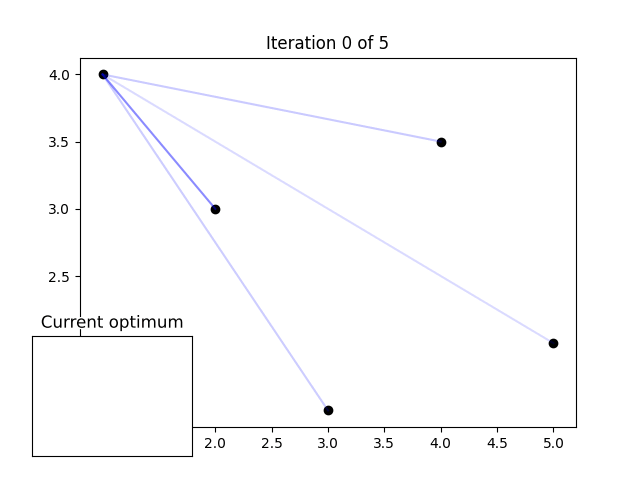Genetic Algorithms

ACO as a solution to the traveling salesman problem

##### Genetic Algorithms
###### ​

Genetic algorithms are based on biological natural selection, the underlying process behind evolution. A population of agents are generated which perform a set of actions corresponding to their ‘genetics’, a set of traits unique to each individual, these agents have defined goals and metrics of success. The more successful an agent is in achieving the desired result, the more likely its genes are to be copied into the next generation. In the context of pathfinding, this generally involves agents who traverse the given graph with the lowest energy spent, or most resources acquired passing their genes on to the next generation.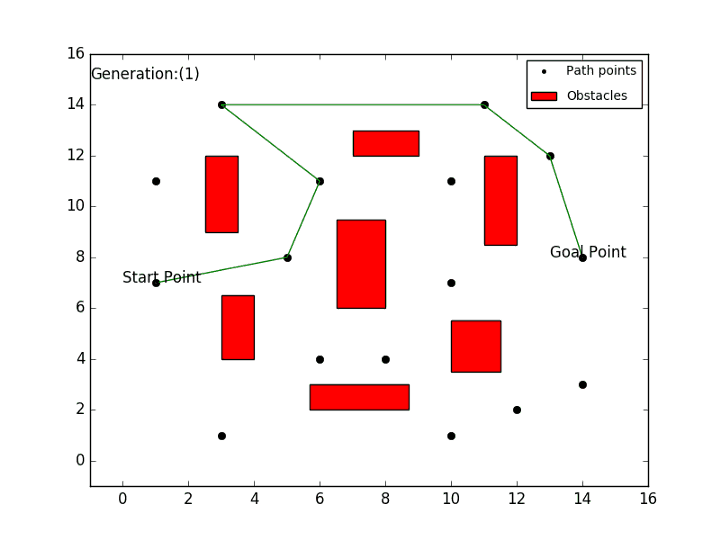Representing Smart Order Routing for DEX Aggregation as a Pathfinding Problem

A genetic algorithm solving a pathfinding problem, source: https://github.com/Yaaximus/genetic-algorithm-path-planning

#### Representing Smart Order Routing for DEX Aggregation as a Pathfinding Problem

In order to understand why pathfinding is a useful concept when devising DEX aggregator SOR algorithms, it is important to remember that a DEX, and consequently a DEX aggregator, is essentially a collection of liquidity pools. These pools act as a point between a pair of assets at which they can be swapped. The following outlines an example in which a DEX is depicted as a graph, over which a pathfinding algorithm is run in order to make a saving on a trade.

###### DISCLAIMER: THIS IS A HYPOTHETICAL, DEMONSTRATIVE EXAMPLE OF HOW SOR CAN BE TREATED AS A PATHFINDING PROBLEM

We can consider this sea of liquidity pools as a graph, in which the pools are nodes, and the possible swaps between them are edges. We can continue this and consider it as a weighted graph, where the weights may represent the profitability or viability of swapping between two pools. For example, consider the following fictional, relatively DAI-centric DEX consisting of 6 liquidity pools (ETH-WBTC, DAI-WBTC, ETH-DAI, DAI-USDT, DAI-FTM, USDT-FTM).Immediately we can see that there does exist a pool such that our swap could be completed, the ETH-DAI pool, however, let’s explore why this may not be the best approach. At each of these nodes, one of the listed tokens can be swapped for the other, in either direction. This means that after swapping at one pool, the other asset is now possessed, as such, after trading with a pool, the new asset can be traded with any pools which also contain that asset. We will demonstrate this relationship by created a directed graph of potential swap sequences.Now let’s consider a scenario in which a trader wishes to swap 100 ETH for as much DAI as possible. We will mark any pools containing ETH as green source nodes at which the path begins, and any pools containing DAI as orange destination nodes at which the path can end, blue nodes denote pools that are neither sources nor destinations.Note that the ETH-DAI pool is a gradient of green and orange, as the path can both begin and end here. Additionally, source nodes have gained a loop that connects this node to itself, this helps in both illustrating the fact that this is also a potential path (representative of the initial swap required to enter the graph), and in the calculations of our pathfinding algorithms.

Next, we assign weights to the edges connecting the nodes. We can think of this as the expected slippage percentage of trading in the pool being pointed at, and we can assume that this is calculated via a variety of metrics that factor in liquidity concentration, liquidity spread, volatility, etc. — you can read more about what causes slippage in our article on smart order routing. It may be the case that these edges require machine learning techniques in order to calculate, some techniques for doing so are explored in our article on machine learning techniques for DEX aggregation and SOR, but for the purposes of this explanation, the numbers have been arbitrarily selected.Note that the swapping of WBTC for DAI is assigned a negative weight, indicating a potential for a 0.2% profit via arbitrage. It is also worth mentioning that algorithms such as Djikstra’s would not be able to handle situations such as this, due to their inability to traverse multi-signed graphs.

We can see that the simplest trade would be to simply use one pool, exchanging ETH for DAI directly, incurring a 5% loss to slippage (5 ETH, or \$10,274.31 USD as of the time of this article’s writing). However, let’s examine our other source node, the ETH-WBTC pool, by identifying the shortest paths between it and all other pools. Any of the previously discussed pathfinding algorithms could handle this task, but in this demonstration we will arbitrarily do so by running the Bellman-Ford algorithm over the graph, starting at the ETH-WBTC pool.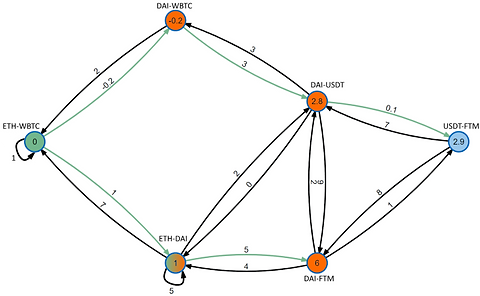References

Green edges indicate the routes from the ETH-WBTC node to all other nodes in the graph. The numbers in the nodes indicate the cost (accumulative percentage losses to slippage) to reach those nodes. Here we can see that by taking advantage of the arbitrage opportunity when trading WBTC for DAI, we can mitigate some of our losses to slippage. Let’s assume our algorithm selects a 70–30 split, transacting 70 ETH first in the ETH-WBTC, then trading that WBTC for DAI, and 70 ETH directly in the ETH-DAI pool.

## 0.3*0.5 + 0.7(0.1–0.02) = 0.206%

This indicates a saving of 4.79%, or approximately \$9,857.24 USD when compared to the simple method of only using one pool. Remembering that these values were arbitrarily selected and that real DEXs stand to lose even more to slippage (see a real example in our article on DEX aggregators).

Furthermore, this approach is merely demonstrative as to how SOR can be thought of as a pathfinding problem, far more complex pathfinding approaches would likely be required, and would likely yield far more impressive results. One reason for the necessity of incorporating more complex pathfinding techniques is the sheer size of graphs relating to a large DEX aggregator. Our example used a hypothetical DEX with only 7 liquidity pools, Real DEXs and DEX aggregators may be dealing with thousands of nodes, which may result in an exponentially large number of edges. To traverse such graphs with perfect optimality is intractable in the timescales required for a usable service. As such, it may be the case that algorithms that specialize in near optimality (such as ACO or genetic algorithms) may be required in order to keep pace with the complexity requirements.

Additionally, it may be the case that the construction of the graph and its edges is a more complicated task than traversing the graph once created. Selecting valid pools, deciding whether to split orders along with the size and number of those splits, assigning weights.

##### References

1. Harinder Kaur Sidhu, University of Windsor, “Performance Evaluation of Pathfinding Algorithms”, https://downloads.hindawi.com/journals/complexity/2021/5511802.pdf, 2021

bottom of page